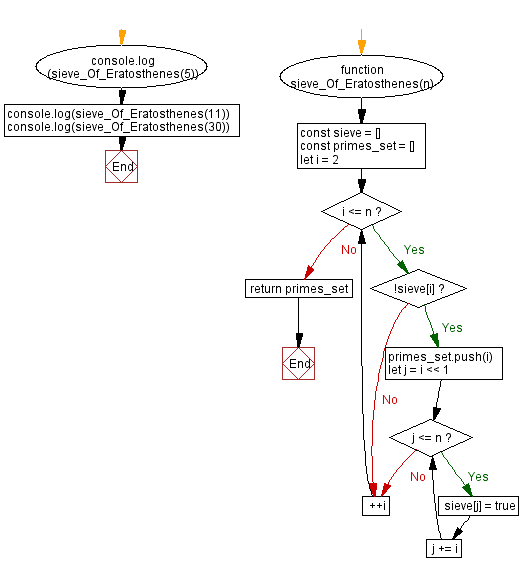# JavaScript: Find all prime numbers below a given number

## JavaScript Math: Exercise-69 with Solution

Write a JavaScript program to find all prime numbers below a given number.

From Wikipedia -
In mathematics, the sieve of Eratosthenes is an ancient algorithm for finding all prime numbers up to any given limit. It does so by iteratively marking as composite (i.e., not prime) the multiples of each prime, starting with the first prime number, 2. The multiples of a given prime are generated as a sequence of numbers starting from that prime, with constant difference between them that is equal to that prime. This is the sieve's key distinction from using trial division to sequentially test each candidate number for divisibility by each prime. Once all the multiples of each discovered prime have been marked as composites, the remaining unmarked numbers are primes.

Sample Data:
(5) -> [2, 3, 5]
(11) -> [2, 3, 5, 7, 11]
(30) -> [2, 3, 5, 7, 11, 13, 17, 19, 23, 29]

Sample Solution:

HTML Code:

``````<!DOCTYPE html>
<html>
<meta charset="utf-8">
<title>JavaScript function to find all prime numbers below a given number</title>
<body>

</body>
</html>
```
```

JavaScript Code:

``````function sieve_Of_Eratosthenes(n) {
const sieve = []
const primes_set = []
for (let i = 2; i <= n; ++i) {
if (!sieve[i])
{
primes_set.push(i)
for (let j = i << 1; j <= n; j += i)
{
sieve[j] = true
}
}
}
return primes_set
}
console.log(sieve_Of_Eratosthenes(5))
console.log(sieve_Of_Eratosthenes(11))
console.log(sieve_Of_Eratosthenes(30))
```
```

Sample Output:

```// [object Array] (3)
[2,3,5]
// [object Array] (5)
[2,3,5,7,11]
// [object Array] (10)
[2,3,5,7,11,13,17,19,23,29]
```

Flowchart:Live Demo:

See the Pen javascript-math-exercise-69 by w3resource (@w3resource) on CodePen.

Improve this sample solution and post your code through Disqus

What is the difficulty level of this exercise?

Test your Programming skills with w3resource's quiz.

﻿